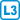## Grid points

The grid points in the plane are colored with 2015 colors. Show that you can choose 2014 rows and 2016 columns so that all their intersections have the same color.

(Grid points are points that have both integer coordinates. The row is made up of points with the same $$y$$ coordinate, and similarly the column for the $$x$$ coordinate.)

• #### Hint

Consider $$n$$ rows for $$n$$ large enough. Assign to each column a $$n$$-tuple of colors corresponding to the intersections with the selected rows. And find columns with the same $$n$$-tuples.

• #### Solution

If we select $$n = 2015\,\cdot\,2013 + 1$$ rows, then according to Dirichlet’s principle, at least one of the 2015 colors will appear 2014 times in each column.

Now take the $$m = 2015^n \cdot 2015 + 1$$ columns. Each column specifies an ordered $$n$$-tuple of the colors used. With the selected number of columns, it is again possible to determine 2016 columns with the same $$n$$ colors according to the Dirichlet’s principle.

Depending on the $$n$$-tuple of colors, it is already easy to select the required 2014 lines.